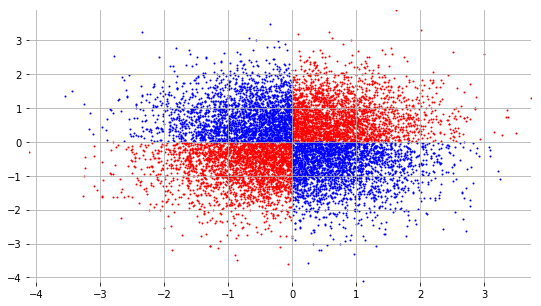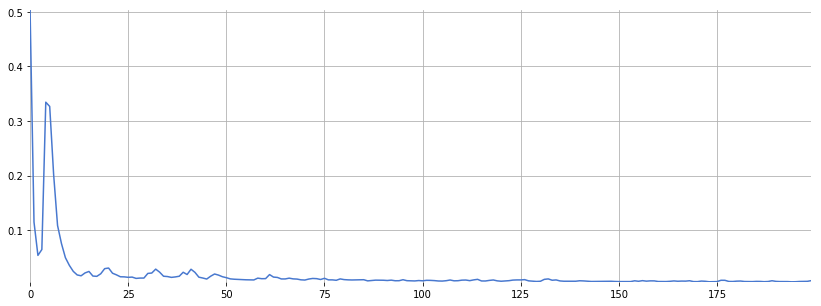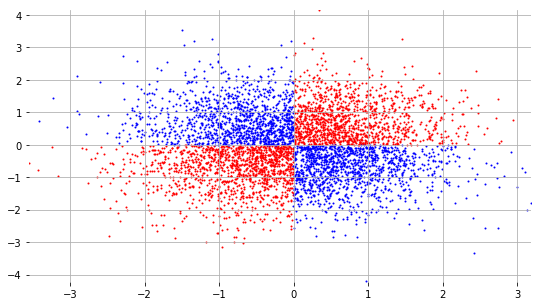# Neural Networks¶

Neural networks are a category of machine learning models which have seen a resurgence since 2006. Deep learning is the recent area of machine learning which combines many neuron layers (e.g. 20, 50, or more) to form a "deep" neural network. In doing so, a deep neural network can accomplish sophisticated classification tasks that classical machine learning models would find difficult.

## Keras¶

Keras is a Python package for deep learning which provides an easy-to-use layer of abstraction on top of Theano and Tensorflow.

Import Keras objects:

In :
from keras.models import Sequential
from keras.layers.core import Dense
import keras.optimizers


Create a neural network architecture by layering neurons. Define the number of neurons in each layer and their activation functions:

In :
model = Sequential()


Choose the optimizer, i.e. the update rule that the neural network will use to train:

In :
optimizer = keras.optimizers.SGD(decay=0.001, momentum=0.99)


Compile the model, i.e. create the low-level code that the CPU or GPU will actually use for its calculations during training and testing:

In :
model.compile(loss='binary_crossentropy', optimizer=optimizer)


## Example: XOR¶

The operation XOR is defined as: XOR(x, y) = 1 if x != y else 0

Synthesize training data for the XOR problem.

In :
X_train = numpy.random.randn(10000, 2)
print(X_train.shape)

(10000, 2)

In :
print(X_train[:5])

[[ 0.42128999  0.41729839]
[ 0.41792333  1.47336136]
[ 0.42125759 -2.28450677]
[ 1.08729453  0.51543248]
[-1.11614025  0.97745119]]


Create target labels for the training data.

In :
y_train = numpy.array([
[float(x*x > 0), float(x*x <= 0)]
for x in X_train
])
print(y_train.shape)

(10000, 2)

In :
y_train[:5]

Out:
array([[1., 0.],
[1., 0.],
[0., 1.],
[1., 0.],
[0., 1.]])

Plot the training data:

Out:
<matplotlib.collections.PathCollection at 0x11b9c6588>Finally, train the model!

In [ ]:
results = model.fit(X_train, y_train, epochs=200, batch_size=100)


Plot the loss function as a function of the training iteration number:

In :
plt.plot(results.history['loss'])

Out:
[<matplotlib.lines.Line2D at 0x11c00a518>]Create test data:

In :
X_test = numpy.random.randn(5000, 2)


Use the trained neural network to make predictions from the test data:

In :
y_test = model.predict(X_test)

In :
y_test.shape

Out:
(5000, 2)

Let's see if it worked:

Out:
<matplotlib.collections.PathCollection at 0x11be77d68>